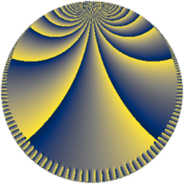# Properties

 Label 252.9.hLevel $252$ Weight $9$ Character orbit 252.h Rep. character $\chi_{252}(251,\cdot)$ Character field $\Q$ Dimension $128$ Sturm bound $432$

# Related objects

## Defining parameters

 Level: $$N$$ $$=$$ $$252 = 2^{2} \cdot 3^{2} \cdot 7$$ Weight: $$k$$ $$=$$ $$9$$ Character orbit: $$[\chi]$$ $$=$$ 252.h (of order $$2$$ and degree $$1$$) Character conductor: $$\operatorname{cond}(\chi)$$ $$=$$ $$84$$ Character field: $$\Q$$ Sturm bound: $$432$$

## Dimensions

The following table gives the dimensions of various subspaces of $$M_{9}(252, [\chi])$$.

Total New Old
Modular forms 392 128 264
Cusp forms 376 128 248
Eisenstein series 16 0 16

## Trace form

 $$128 q + O(q^{10})$$ $$128 q + 55676 q^{16} + 1139852 q^{22} + 11274752 q^{25} + 153140 q^{28} - 8577920 q^{37} - 883116 q^{46} - 18969088 q^{49} + 4666324 q^{58} + 64778880 q^{64} + 30349680 q^{70} + 69619072 q^{85} - 425093084 q^{88} + O(q^{100})$$

## Decomposition of $$S_{9}^{\mathrm{new}}(252, [\chi])$$ into newform subspaces

The newforms in this space have not yet been added to the LMFDB.

## Decomposition of $$S_{9}^{\mathrm{old}}(252, [\chi])$$ into lower level spaces

$$S_{9}^{\mathrm{old}}(252, [\chi]) \cong$$ $$S_{9}^{\mathrm{new}}(84, [\chi])$$$$^{\oplus 2}$$